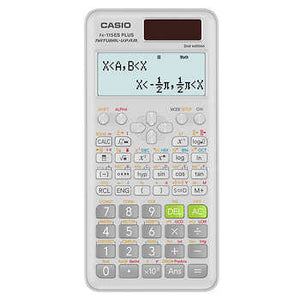¡Con un solo clic todo llega a ti!Español es
•English en
•Español es
¡Con un solo clic todo llega a ti!Español es
•English en
•Español es
Carrito 0# Casio 2nd Edition Scientific Calculator FX-115ESPLS2-S

Precio habitual \$19.99 \$0.00 Precio unitario por
Los gastos de envío se calculan en la pantalla de pagos.
Casio FX-115ESPLS2-S 2nd Edition Scientific Calculator with slide on hard case and sleek new design. With the Natural Textbook Display, in addition to advance Statistics, Matrix, and Conversion functionality, is a perfect choice for high school and college students. Recommended for students taking General Math, Pre-Algebra, Algebra I and II, Geometry, Trigonometry, Statistics, Calculus, Chemistry, Engineering.

• Accessories Included: Slide-On Hard Protective Case
• Matrix and Vector Calculations: The Matrix mode allows you to perform matrix operations on matrices with up to 3 rows and 3 columns. It also allows you to enter vectors and perform vector calculations.
• Simultaneous and Polynomial Solver: This calculator will solve simultaneous linear equations with two unknowns, simultaneous linear equations with three unknowns, quadratic equations and cubic equations.
• Complex Number Calculations: Your calculator can perform the following: Addition, subtraction, multiplication, division. Augment and absolute value calculations. Reciprocal, square and cube calculations. Conjugate complex number calculations.
• Statistics and Linear Regression: This calculator can linear regression, quadratic regression, logarithmic regression, exponential regression, ab exponential regression, power regression and inverse regression.
• Converts between Standard form and a Decimal: This calculator can transform a displayed decimal calculation result to a fraction or mixed number. It can also be converted back to the decimal form.
• 2-line Natural Textbook Display shows formula and results exactly as they appear in the textbook and displays formulas and results simultaneously for 417 built-in functions
• Fraction calculations. Fraction/decimal conversions, simplifying fractions and roots, recurring decimal display, remainder calculation, integer division with remainder
• Prime factorization
• Metric conversion
• Hyperbolic functions
• Greatest common divisor and least common multiple
• Random Integer generator
• Summation notation
• Verify Computations